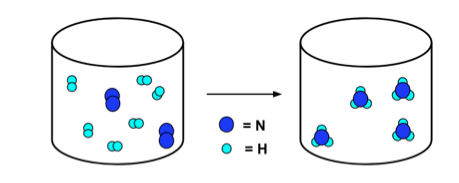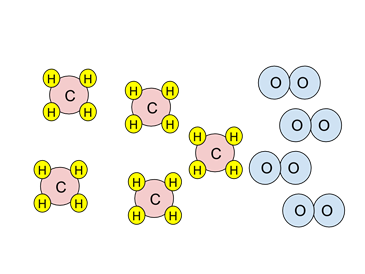# Enduring Understanding 1.E: Conservation of Matter and Gravimetric Analysis

• Atoms are never created or destroyed in physical and chemical processes. This is sometimes called the 'conservation of matter' or 'conservation of mass'. An exception to this is certain radiochemical processes.
• Reactions can be illustrated by equations and by particulate diagrams. Consider the reaction:
• N2 + 3H2 → 2NH3
• The particulate diagram below illustrates this reaction. Note that the number of nitrogen atoms (dark blue) and hydrogen atoms (light blue) are the same on the left and right of the arrow.
•• Because atoms are neither created nor destroyed, but are conserved in chemical reactions, the amount of a product formed in a chemical reaction can be measured to determine the amount of the reactant(s) that were originally present.

• An example of this is gravimetric analysis. In gravimetric analysis reactants form a precipitate, which is then weighed to determine the amount of reactant originally present. To solve a gravimetric analysis problem:
• Use the grams of precipitate to find the moles of precipitate (mass/molar mass)
• Use the balanced equation to calculate the moles of solute.
• Use the volume of the original solution to calculate the concentration (moles/volume)

• Sample Problem: 25.00 mL of a lead (II) nitrate (Pb(NO3)2) solution is treated with excess aqueous sodium sulfate (Na2SO4). After filtration and drying, 0.303 g of solid lead sulfate (PbSO4) is isolated. What was the concentration of the lead (II) nitrate solution? The molar mass of lead sulfate is 303.2 g/mol

• The balanced equation is Pb(NO3)2 + Na2SO4 → PbSO4(s) + 2 NaNO3
• First, the moles of precipitate formed is 0.303 g/303.2 g/mol or 1.00 x 10-3 moles.
• The coefficients in the chemical equation are 1 for both Pb(NO3) and PbSO4. So the number of moles of lead nitrate originally present is 1.00 x 10-3 moles.
• The original concentration is 1.00 x 10-3 mol / 0.02500 L or 0.0400 mol/L.
• The concentration of the lead nitrate solution was 0.0400 mol/L.

• Another type of analysis is volumetric analysis, often called titration. Titration finds the concentration of an unknown reactant in solution by adding a measured amount of a species ('titrant') that reacts with the reactant ('analyte'). When a sufficient amount of the reacting species has been added, a color or some other change occurs and the concentration of the unknown can be determined. To solve a titration problem:
• Determine the number of moles of titrant added.
• Use the balanced equation to determine the number of moles of analyte present.
• Use the volume of the original solution to calculate the concentration (moles/volume)

• Sample Problem: 25.00 mL of a bydrobromic acid (HBr) solution was titrated with 41.9 mL of 0.352 mol/L sodium hydroxide (NaOH) solution. What is the concentration of the HBr solution?

• The balanced equation is HBr(aq) + NaOH (aq) → NaBr (aq) + H2O
• Number of moles of sodium hydroxide added: 0.0419L x 0.352 mol/L = 0.0147 mol NaOH
• The coefficients in the chemical equation are 1 for HBr and NaOH, so the amount of HBr originally present must be 0.0147 mol HBr.
• The HBr concentration must be 0.0147 mol/0.02500 L = 0.590 mol/L.

• Often chemical reaction problems will be presented as limiting reagent problems. Because atoms and molecules react in definite and fixed proportions, sometimes there will be too much of one reagent for that reagent to be completely consumed.

• Example: Consider the particulate diagram below. If the combustion reaction went to completion, what species would be present after the combustion?
•• The reaction is the combustion of methane, CH4:
• CH4 + 2O2 → CO2 + 2H2O
• Look at the stoichiometry of the reaction. Two oxygen molecules (in blue) are needed to react with one methane molecule (red & yellow).
• There are four oxygen molecules. Since two are needed to react with one methane, there is only enough oxygen to react with two methanes. Oxygen is the limiting reagent.
• When the combustion has taken place, two methanes and all four oxygens will be consumed. Three methanes will not have reacted; they are the excess reagent.
• So at the end of the reaction there would be two CO2s, four H2Os, and three unreacted CH4s.

 Related Links: Chemistry Chemistry Quizzes AP Chemistry Notes Experimental Data and Atomic Structure AP Chemistry Quizzes Atoms, Molecules, and Chemical Analysis Quiz Electronic Structure of Atoms Quiz Periodicity, Atomic & Ionic Radii, Atomic Models Quiz Conservation of Matter and Balanced Equations Quiz Gases and Solutions Quiz Intermolecular Forces Quiz Molecular Geometry and VSEPR Quiz Bonding and Solid State Properties Quiz Balanced and Ionic Equations Quiz Chemical Reactions Quiz Redox Reactions and Electrochemistry Quiz Chemical Kinetics and Rates Quiz Reaction Rates and Collisions Quiz Multi-Step Reaction Rates and Catalysis Quiz Energy and Heat Quiz Conservation of Energy and Calorimetry Quiz Bond Energies Quiz Entropy and Gibbs Free Energy Quiz Chemical Equilibrium Quiz Solubility and Acid-Base Equilibrium Quiz

To link to this Conservation of Matter and Gravimetric Analysis page, copy the following code to your site: